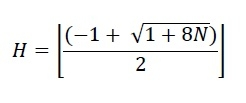# C/C++ Program for Maximum height when coins are arranged in a triangle?

In this section, we will see one interesting problem. There are N coins. we have to find what is the max height we can make if we arrange the coins as pyramid. In this fashion, the first row will hold 1 coin, second will hold 2 coins and so on.In the given diagram, we can see to make a pyramid of height three we need minimum 6 coins. We cannot make height 4 until we have 10 coins. Now let us see how to check the maximum height.

We can get the height by using this formula.## Example

Live Demo

#include<iostream>
#include<cmath>
using namespace std;
int getMaxHeight(int n) {
int height = (-1 + sqrt(1 + 8 * n)) / 2;
return height;
}
main() {
int N;
cout << "Enter number of coins: " ;
cin >> N;
cout << "Height of pyramid: " << getMaxHeight(N);
}

## Output

Enter number of coins: 13
Height of pyramid: 4